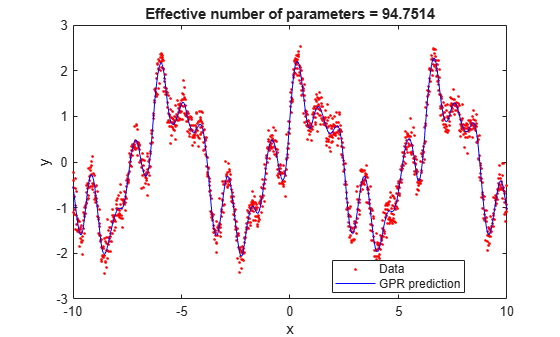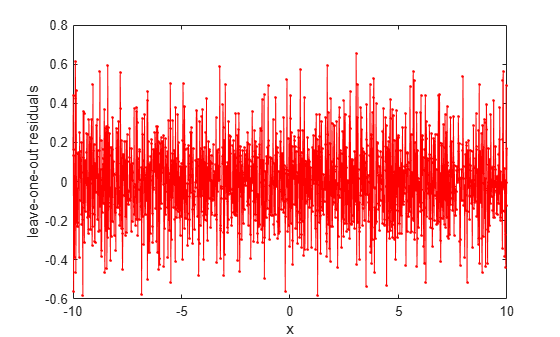# postFitStatistics

Class: RegressionGP

Compute post-fit statistics for the exact Gaussian process regression model

## Syntax

```loores = postFitStatistics(gprMdl) [loores,neff] = postFitStatistics(gprMdl) ```

## Description

`loores = postFitStatistics(gprMdl)` returns the leave-one-out residuals, `loores`, for the trained Gaussian process regression (GPR) model.

`[loores,neff] = postFitStatistics(gprMdl)` also returns the number of effective parameters, `neff`.

## Input Arguments

expand all

Gaussian process regression model, specified as a `RegressionGP` object.

## Output Arguments

expand all

Leave-one-out residuals, returned as an n-by-1 matrix, where n is the number of observations in the training data.

Number of effective parameters, returned as an n-by-1 matrix, where n is the number of observations in the training data.

## Examples

expand all

Generate sample data.

```rng(0,'twister'); % For reproducibility n = 1500; x = linspace(-10,10,n)'; y = sin(3*x).*cos(3*x) + sin(2*x).*cos(2*x) + sin(x) + cos(x) + 0.2*randn(n,1);```

Fit a GPR model using the exact method for fitting and prediction.

```gprMdl = fitrgp(x,y,'Basis','linear','FitMethod','exact',... 'PredictMethod','exact','KernelFunction','matern52');```

Compute the leave-one-out residuals and the effective number of parameters in the trained model.

`[loores,neff] = postFitStatistics(gprMdl);`

Predict the responses using the trained model.

`ypred = resubPredict(gprMdl);`

Plot the true and predicted responses, and display effective number of parameters in the fit.

```figure() plot(x,y,'r.'); hold on; plot(x,ypred,'b'); xlabel('x'); ylabel('y'); legend('Data','GPR prediction','Location','Best'); title(['Effective number of parameters = ',num2str(neff)]); hold off```Plot leave-one-out residuals.

```figure() plot(x,loores,'r.-'); xlabel('x'); ylabel('leave-one-out residuals');```## Tips

• You can only compute the post-fit statistics when `PredictMethod` is `'exact'`.

• If `FitMethod` is `'exact'`, then `postFitStatistics` accounts for the fact that the fixed basis function coefficients are estimated from the data.

• If `FitMethod` is different than `'exact'`, then `postFitStatistics` treats the fixed basis function coefficients as known.

• For all `PredictMethod` and `FitMethod` options, `postFitStatistics` treats the estimated kernel parameters and noise standard deviation as known.

## Version History

Introduced in R2015b